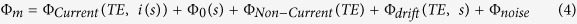## ΜRI quantification of magnetic field changes with MRCDI and MREIT

Magnetic resonance current density imaging (MRCDI) and MR electrical impedance tomography (MREIT) are two emerging techniques that combine current injection with magnetic resonance to determine the current density of the human brain.

#### Brain Magnetic Resonance Current Density Imaging

In magnetic resonance imaging (MRI), the static magnetic field of the scanner, conventionally on the z axis, aligns the spins of the subject resulting in a net longitudinal magnetization, represented by vector Mz (z axis). In MRICDI (Göksu et al 2018), an applied current induces a magnetic field in the head and the induced magnetic field component in the z axis, termed ΔB(c)z, causes small shifts in the precession frequency of the magnetization vector given that this frequency depends on magnetic field intensity. This introduces either an acceleration or a deceleration (lag) of the precession, or in other words it changes the phase and specifically, it modulates the phase of the measured MRI signal proportionally to ΔB(c)z. The measurement of the current-induced phase changes can be used to determine the induced ΔB(c)z and to reconstruct the inner current flow and the conductivity distribution.

The technique makes use of MRI sequences i.e. sets of pulses and gradients such as multi-echo spin echo (MESE) and steady-state free precession free induction decay (SSFP-FID), with the purpose of generating an optimal signal (Göksu et al 2018). Representative images of SSFP-FID measurements with multi-gradient-echo readouts performed in five subjects using three different repetition times TR are shown in Figure 10 (Göksu et al 2018) where (a) shows ΔΒz(c) without current injection and (b) demonstrates ΔΒz(c) with current injection.

A current flow reconstruction algorithm based on first order spatial derivatives of the measured current-induced magnetic field is used by Göksu et al 2018. It is assumed that the x and y components can be neglected.

Figure 10: Experiment 4 (Göksu et al 2018): Comparison of SSFP-FID measurements with multi-gradient-echo readouts performed in five subjects using three different repetition times TR. (a) ΔΒz(c) without current injection. (b) ΔΒz(c) with current injection

#### ΜRI quantification of magnetic field changes induced by tDCS* current in human brain

Magnetic resonance imaging has been also used for quantification of magnetic field changes induced by tDCS current in the human brain (Jog MV et al 2016). As mentioned by the authors at the supplemental material (Jog MV et al 2016), the magnetic field of the brain perturbs the magnetic field of the scanner or in other words it introduces a perturbation in the magnetic field of the scanner. Any perturbation of the magnetic field ΔB (vector) can be written as the sum of two orthogonal components, one along Bz (parallel to Bz), and the other perpendicular to Bz. The total magnetic field can be written as the vector sum of Bz and the perturbation or equivalently, as a vector of length Bmag, at an angle θ to Bz. For perturbations on the order of ppm, it is proven that θ is almost zero i.e. Btotal is along Bz.

Using MRI field mapping, field variations along Bz can be measured as phase angles according to:

Φm = (γ *ΔBz * TE) mod(2π)

where Φm is the measured phase angle between 0 and 2π radians, γ is the gyromagnetic ratio, ΔBz is the field deviation along Bz and TE is the echo time. The results show that it is possible to detect magnetic field changes as small as a nanotesla (nT) with a spatial resolution of a few millimeters.

The above equation can be written as ΔBz = ΔΦm / γ x ΔTE as mentioned in the Supplement (Jog MV et al 2016).

The modulo-2π operation has been removed indicating that the phase angles have been unwrapped. Δ represents the fact that we are referring to the phase difference between two TE’s. In an ideal scenario without noise, the minimum (unbiased) detectable field corresponds to the smallest possible non zero phase change generated by the smallest applied current (0.5mA). Because the full phase range of 0 to 2π radians is divided into 4096 discrete levels in MRI, the smallest non-zero phase change evaluates to 2π/4096 radians. For a given ΔTE of 9.84 msec, the minimum (unbiased) detectable field equates to 0.58nT at 0.5mA, or ~ 1.2nT/mA. It is possible to increase the ΔTE by minimizing TE1, or, increasing TE2 permitted by SNR.

It is noted that in General Linear Model Analysis the measured phase was modeled as:#### ΜRI quantification of magnetic field changes induced by tACS* current in human brain using magnetic resonance electrical impedance tomography (MREIT)

Magnetic resonance electrical impedance tomography (MREIT) has been used for quantification of magnetic field changes induced by tACS* current in human brain (Kasinadhuni AK et al 2017).